output.to from Sideway
Draft for Information Only

# Content

``` Centroid by Geometric Decomposition   Centroids of Composite Plane Body   Centroid of composite plane plate 1   Centroid of composite plane plate 2   Centroid of Composite Wire```

# Centroid by Geometric Decomposition

By denoting the position of the element of a plane body relative to the coordinate system as xi,yi, the coordinates x, y ,of the center of gravity of the plane body where the resultant force W applied can be determined by equating the moment of the resultant force W about the y and x axes and the corresponding mements of elements of the plane body about the coordinate axis respectively. Imply.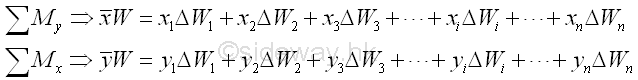Therefore the coordinates x , y of the center of gravity of the plane body is.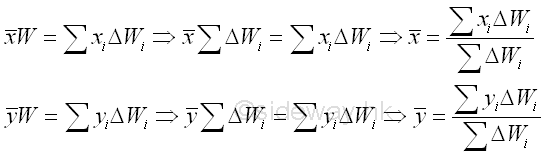If a 2D plane body can be divided into some common plane bodys, the 2D plane body can be considered as a composite 2D plane body. For a composite 2D plane body, the cooresponding mements of elements of the plane body can also be grouped according to the composition of the 2D plane body. ImplyFor a homogeneous 2D plane plate of uniform thickness, the first moment of the 2D plane plate area is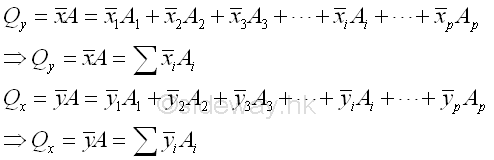For a homogeneous 2D plane wire of uniform area, the first moment of the 2D plane wire fragement is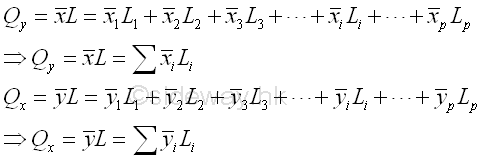## Centroids of Composite Plane Body

### Centroid of composite plane plate 1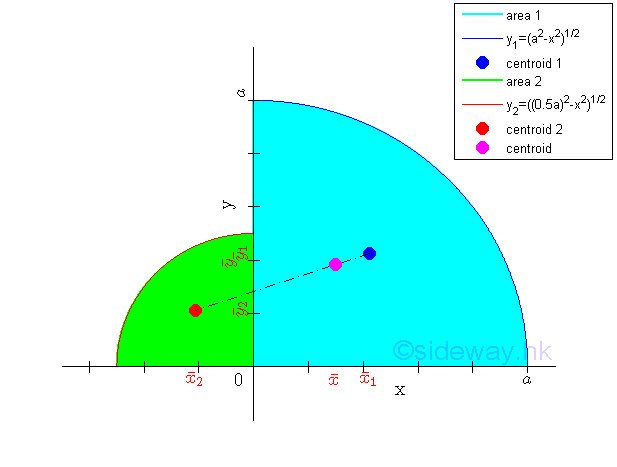For example, the signed area of a composite planar region R bounded by curves in rectangular form , Imply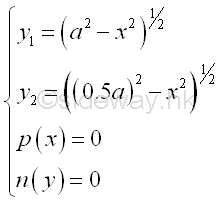The planar region R can be divided into two common shapes. Therefore, centroid of the bounded area by concept of composite plane body is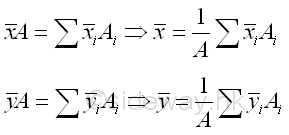The area of the planar region isCoordinate x of centroid

The first moment of area can be either positive and negative. The centroid of area 1 is located to the right of the y axis, the first moment of area is positive.  Since the centroid of area 2 is located to the left of the y axis, the first moment of area is negative and the negative sign is usually assigned to the x coordinate of the centroid of area 2. Imply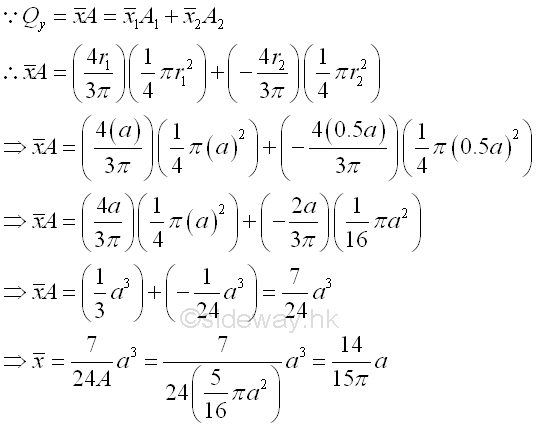The coordinate x of centroid of area of the region is positive also implies that the coordinate x of the centroid is located to the right of y axis.

Similarly, coordinate y of centroid

Since both the centroid of area 1 and the centroid of area 2 are located above the x axis, the first moment of both area 1 and area 2 should be positive. Imply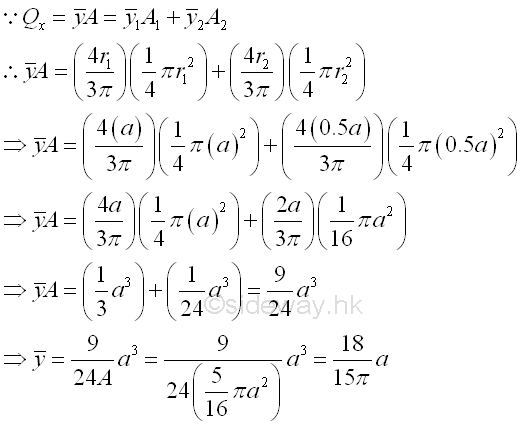The coordinate y of centroid of area of the region is positive also implies that the coordinate y of the centroid is located above x axis.

For a region with two common shapes, area 1 and area 2, the centroid of area of the region is located on the line joining the centroids of the area 1 and area 2. And the centroid of area of the region lies between the two centroids of the two common shapes.

### Centroid of composite plane plate 2

Since the first moment of area of a hole can be considered as the negative sense of the first moment of area by assuming the first moment of area of the same area of opposite sense. Therefore the first moment of area of a hole is negative. The negative sign is usually assigned to the area of the hole and the area of a hole is called negative area.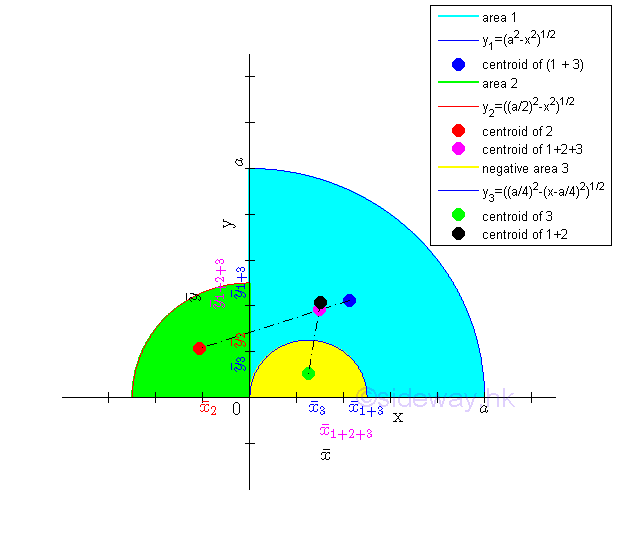For example, the signed area of a composite planar region R bounded by curves in rectangular form , ImplyThe planar region R can be divided into common shapes. Therefore, centroid of the bounded area by concept of composite plane body isThe area of the planar region is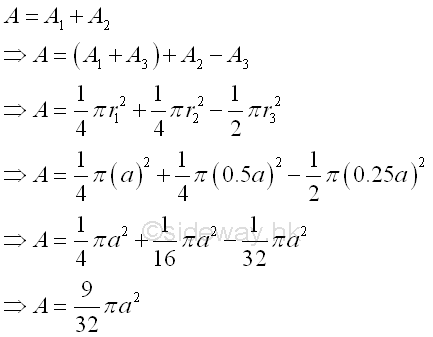Coordinate x of centroid

The first moment of area can be either positive and negative. The centroid of area 1 plus area 3 is located to the right of the y axis, the first moment of area is positive.  Since the centroid of area 2 is located to the left of the y axis, the first moment of area is negative and the negative sign is usually assigned to the x coordinate of the centroid of area 1. And for the centroid of hole, negative area 3 is located to the right of the y axis, the coordinate of the centroid of area 3 is positive but the sense of the area is negative. Imply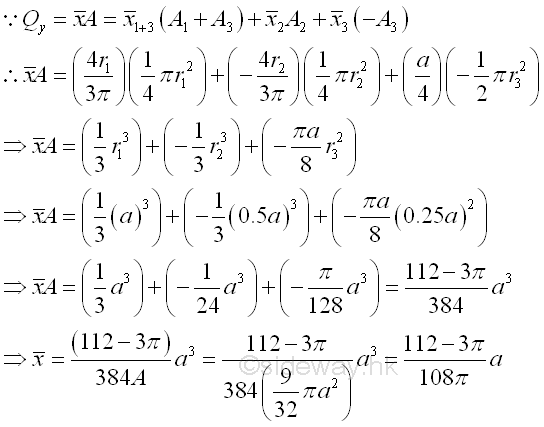The coordinate x of centroid of area of the region is positive also implies that the coordinate x of the centroid is located to the right of y axis.

Similarly, coordinate y of centroid

Since both the centroid of area 1 and the centroid of area 2 are located above the x axis, the first moment of both area 1 and area 2 should be positive. And for the centroid of hole, negative area 3 is located above the x axis, the coordinate of the centroid of area 3 is positive but the sense of the area is negative. Imply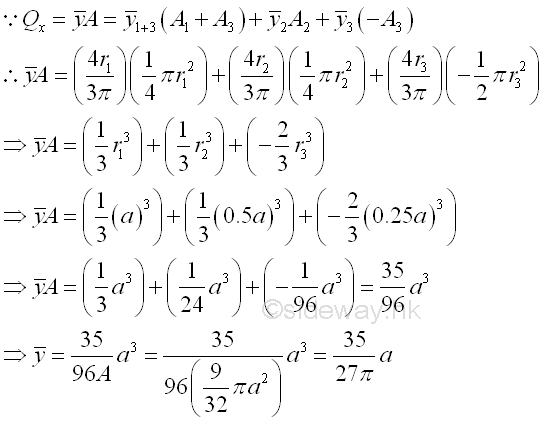The coordinate y of centroid of area of the region is positive also implies that the coordinate y of the centroid is located above x axis.

For a region of two common shapes, area 1+2+3 and negative area 3, the centroid of area of the region is located on the line passing through the centroids of the area 1+2+3 and negative area 3. And because of the the negative sense of the hole, negative area 3, the centroid of area of the region lies outside the two known centroids of the two shapes.

### Centroid of Composite Wire

Centroid of a composite wire can be determined in similar way.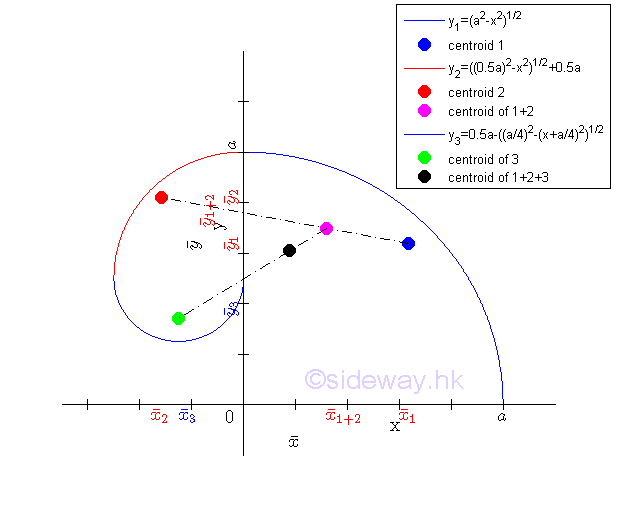.

For example, the composite wire fragment L bounded by curves in rectangular form , Imply.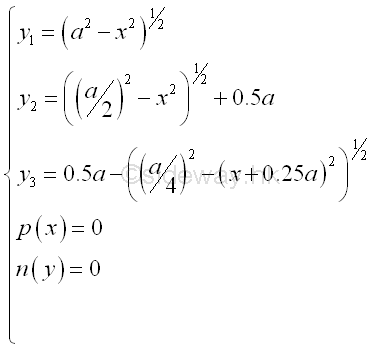The planar fragment L can be divided into common shapes. Therefore, centroid of the bounded wire fragment by concept of composite plane body is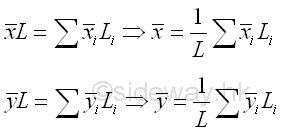The length of the planar fragment isCoordinate x of centroid

The first moment of length can be either positive and negative also. The centroid of length 1 is located to the right of the y axis, the first moment of length is positive.  Since the centroid of length 2 and length 3 is located to the left of the y axis, the first moment of length is negative and the negative sign is usually assigned to the x coordinate of the centroid of length. Imply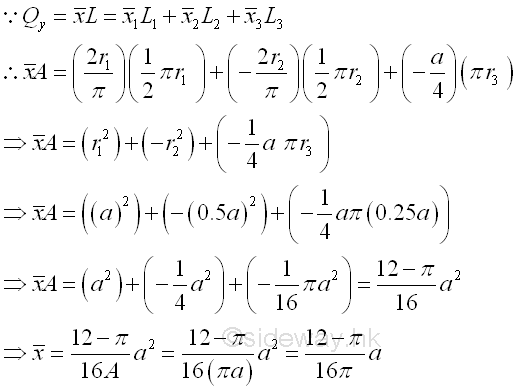The coordinate x of centroid of length of the curve fragement is positive also implies that the coordinate x of the centroid is located to the right of y axis.

Similarly, coordinate y of centroid

Since both the centroid of length 1, length 2 and length 3 are located above the x axis, the first moment of length of length 1, length 2 and length 3 should be positive. Imply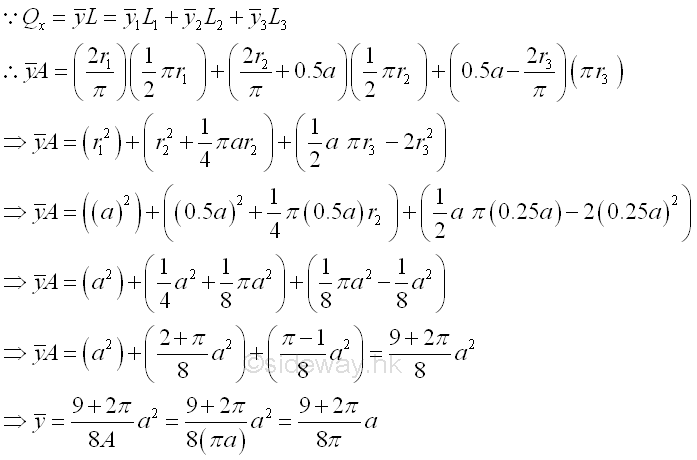The coordinate y of centroid of length of the curve fragement is positive also implies that the coordinate y of the centroid is located above x axis.

For a curve fragment with two common shapes, length 1 and length 2, the centroid of lengthof the curve fragment is located on the line joining the centroids of the length 1 and length 2. The centroid of length of the curve fragement lies between the two centroids of the two common shapes.  And the centroid of the whole curve fragment can be determined similarly by starting with any pair of the common shapes of the curve..

ID: 120600007 Last Updated: 11/6/2012 Revision: 0 Ref:References

1. I.C. Jong; B.G. rogers, 1991
2. F.P. Beer; E.R. Johnston,Jr.; E.R. Eisenberg, 2004Home 5

Management

HBR 3

Information

Recreation

Culture

Chinese 1097

English 337

Computer

Hardware 149

Software

Application 187

Numeric 19

Programming

Web 761

CSS 1

HTML

Knowledge Base

OS 389

MS Windows

Knowledge

Mathematics

Algebra 20

Geometry 18

Calculus 67

Engineering

Mechanical

Rigid Bodies

Statics 92

Dynamics 37

Control

Physics

Electric 11